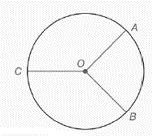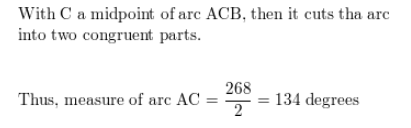# a) If m A B ⌢ = 88 ° , then m A C B ⌢ = ____________. b) If m A B ⌢ = 92 ° and C is the midpoint of major are ACB, then m A C ⌢ = _____________.### Elementary Geometry for College St...

6th Edition
Daniel C. Alexander + 1 other
Publisher: Cengage Learning
ISBN: 9781285195698### Elementary Geometry for College St...

6th Edition
Daniel C. Alexander + 1 other
Publisher: Cengage Learning
ISBN: 9781285195698

#### Solutions

Chapter
Section
Chapter 6.CT, Problem 1CT
Textbook Problem

## a) If m A B ⌢ = 88 ° , then m A C B ⌢ = ____________.b) If m A B ⌢ = 92 ° and C is the midpoint of major are ACB, then m A C ⌢ = _____________.Expert Solution
To determine

(a)

To find:

The measure of mACB=

Solution:

mACB=272°.

### Explanation of Solution

Given:

mAB=88°

Approach:

The degree measured around a circle is 360°.

Calculation:From the above circle we have,

mACB+mAB=360°mACB+88°=360°mACB=360°88°mACB=272°

Expert Solution
To determine

(b)

To find:

The measure of mAC.

Solution:

mAC=134°.

### Explanation of Solution

Given:

mAB=92°.

C is the midpoint of major are ACB.

Approach:

The degree measured around a circle is 360°.

Calculation:From the above circle we have,

mACB+mAB=360°mACB+92°=360°mACB=360°92°mACB=268°

Now to find the measure of mAC.

It is given that C is the midpoint of major are ACB.C is the midpoint of the arc ACB, then it cuts the arc into two congruent parts.

Thus, measure of the arc is mAC=mACB2.

mAC=2682mAC=134°

### Want to see more full solutions like this?

Subscribe now to access step-by-step solutions to millions of textbook problems written by subject matter experts!

Get Solutions

### Want to see more full solutions like this?

Subscribe now to access step-by-step solutions to millions of textbook problems written by subject matter experts!

Get Solutions